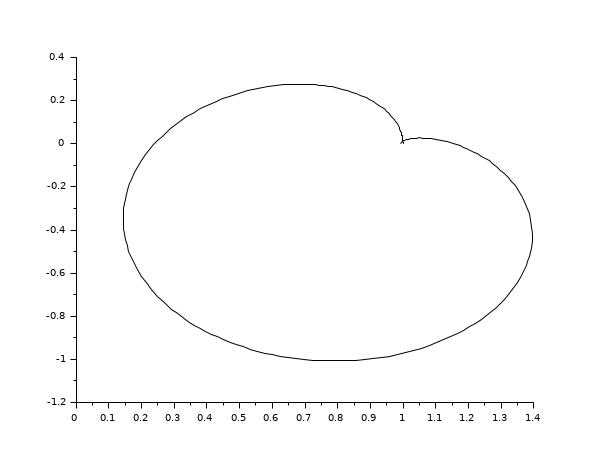Change language to:
Français - 日本語 - Português - Русский

See the recommended documentation of this function

# calfrq

frequency response discretization

### Syntax

`[frq, bnds, split] = calfrq(h, fmin, fmax)`

### Arguments

h

A siso or simo linear dynamical system, in state space, transfer function or zpk representations, in continuous or discrete time.

fmin,fmax

real scalars (min and max frequencies in Hz)

frq

row vector (discretization of the frequency interval)

bnds

vector `[Rmin Rmax Imin Imax]` where `Rmin` and `Rmax` are the lower and upper bounds of the frequency response real part, `Imin` and `Imax` are the lower and upper bounds of the frequency response imaginary part,

split

vector of frq splitting points indexes

### Description

frequency response discretization; `frq` is the discretization of `[fmin,fmax]` such that the peaks in the frequency response are well represented.

Singularities are located between `frq(split(k)-1)` and `frq(split(k))` for `k>1`.

### Examples

```s=poly(0,'s')
h=syslin('c',(s^2+2*0.9*10*s+100)/(s^2+2*0.3*10.1*s+102.01))
h1=h*syslin('c',(s^2+2*0.1*15.1*s+228.01)/(s^2+2*0.9*15*s+225))
[f1,bnds,spl]=calfrq(h1,0.01,1000);
rf=repfreq(h1,f1);
plot2d(real(rf)',imag(rf)')```• bode — Bode plot
• black — Black-Nichols diagram of a linear dynamical system
• nyquist — nyquist plot
• freq — frequency response
• repfreq — frequency response
• logspace — logarithmically spaced vector

### History

 Version Description 6.0 handling zpk representation
 Report an issue << bode_asymp Frequency Domain dbphi >>